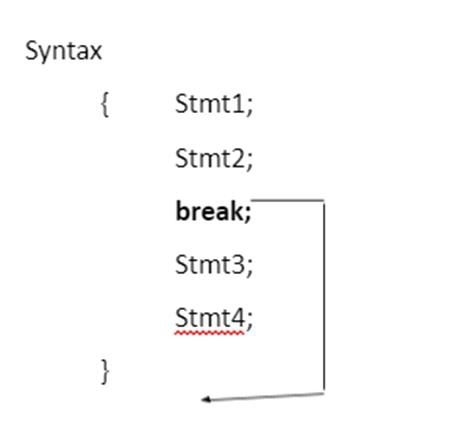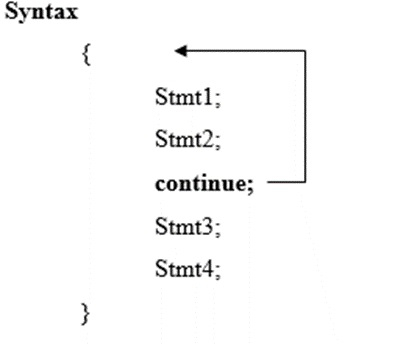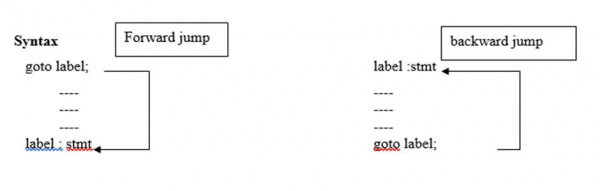# Nested for loop and other related statements in C language

In nested for loops, more than one for statements are included in the body of the loop.

The number of iterations will be equal to number of iterations in the outer loop multiplied by the number of iterations in the inner loop.

## Example

Following is the C program for nested for loop −

Live Demo

#include<stdio.h>
main( ){
int i,j;
for (i=1; i<=2; i++){
for (j=1;j<=2; j++){
printf ("%d", i*j);
}
}
}

## Output

When the above program is executed, it produces the following result −

1 2 3 4 5 6
Execution i*j
i=1 j=1 1
j=2 2
i=2 j=1 2
j=2 4

The other related statements in C language are as follows −

• break
• continue
• goto

## break

• It is a keyword used to terminate the loop (or) exit from the block.

• The control jumps to next statement after the loop (or) block.

• break is used with for, while, do-while and switch statement.

• When break is used in nested loops then only the innermost loop is terminated.## Example

Following is the C program for break statement −

Live Demo

#include<stdio.h>
void main( ){
int i;
for (i=1; i<=5; i++){
printf ("%d", i);
if (i= =3)
break;
}
}

## Output

When the above program is executed, it produces the following result −

1 2 3

## continue

• It is a keyword which is used for continuing the next iteration of the loop.
• It skips the statements after the continue statement.
• It is used with for, while and do-while.## Example

Following is the C program for continue statement −

Live Demo

#include<stdio.h>
void main( ){
int i;
for (i=1; i<=5; i++){
if (i==2)
continue;
printf("%d", i);
}
}

## Output

When the above program is executed, it produces the following result −

1 2 3 4 5

## goto

It is used after the normal sequence of program execution by transferring the control to some other part of program.## Example

Following is the C program for goto statement −

Live Demo

#include<stdio.h>
void main( ){
printf("Hello");
goto l1;
printf("How are");
l1: printf("you");
}

## Output

When the above program is executed, it produces the following result −

Hello
you